Coordinate Transformations in the Vector Analysis Package

It is no surprise that many of these differential operations already exist in Mathematica packages.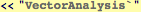Coordinate Systems

Converting between coordinate systems

The spherical coordinates expressed in terms of the cartesian x,y,z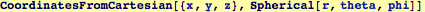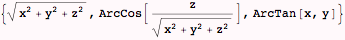The cartesian coordinates expressed in terms of the spherical r θ φ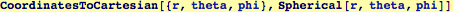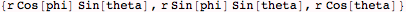The equation of a line  through the origin in spherical coodinates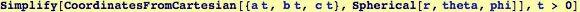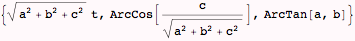Created by Wolfram Mathematica 6.0  (21 September 2007)